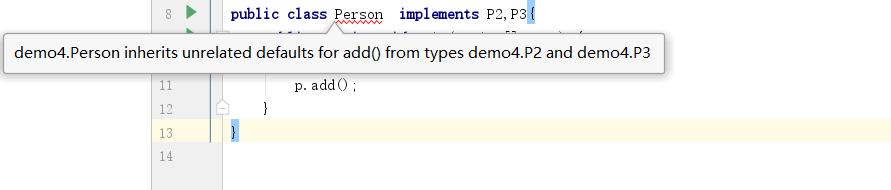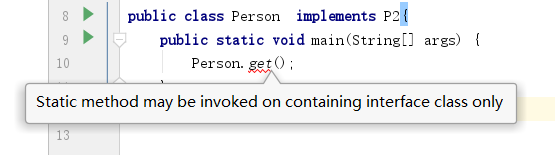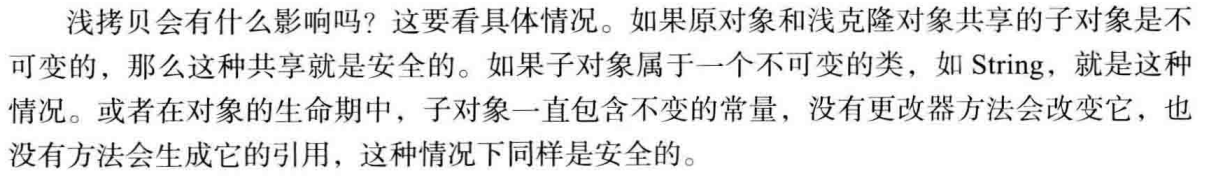# java核心技术(第十版卷一)笔记(纯干货!)

--------------------------------------------------------------------------------------------------------

自动类型转换两个数值.如果有double,则转换成double.否则有float,转换成float.否则有long,转换long.否则都转换成int
类之间的关系依赖(uses-a):A类依赖B类.A类需要调用B类聚合(has-a):A类包含B类.继承(is-a):A类继承自B类.A类包含B类的属性行为,以及有自己的属性和行为
对于方法参数其实是有一个隐藏的参数,就是this.表示当前的调用对象,而静态方法中,则不存在this.因为静态方法不依赖实例对象final修饰类的原则如果一个类的内部,没有提供可修改类本身状态的方法.则可将该类设为final(被final修饰的类不可以被继承,如果你继承了一个final类,则代表你可以自定义方法修改final类的属性,其破坏了封装性.这个坑在某个面试中,被坑了....

如果一个class被声明为final,那么其所有的方法也默认被设置为final,而字段则不在final中.被final修饰的类主要目的还是阻止其他类通过成为其子类的方式而修改本身类的行为.

java的参数统一是按照值传递的.基本数据类型传递的值的副本.引用数据类型传递的引用的副本,所以方法内部对参数进行的修改,不会影响实参.
public class Person {
public int age = 1;
}
public class Test {
public static void main(String[] args) {
int y = 1;
change(1);
System.out.println(y);//1
Person p = new Person();
change(p);
System.out.println(p.age);//2
change2(p);
System.out.println(p.age);//2
}

public static void change(int x) {
x = 2;
}

public static void change(Person p) {
p.age = 2;
}

public static void change2(Person p) {
p = new Person();
p.age = 3;
}
}

equals特性

1 自反性: 对于任何非空引用x,x.equals(x);应当返回true.

2 对称性: 对于任何引用x和y,当且仅当y.equals(x);返回true,x.equals(y);也应当返回true.

3 传递性: 对于任何引用x,y,z如果x.equals(y)返回true; y.equals(z)返回true;那么x.equals(z);应当返回true

4 一致性: 如果x和y的引用对象没有发生变化,返回调用x.equals(y);应当返回同样的结果.

5 对于任意非空引用 x,x.equals(null);应当返回false.

public static void main(String[] args) {
Integer i = 100;
i = i + 1;
System.out.println(i);
}

    public static void main(String[] args) {
Integer i = 1000;
Integer x = 1000;
System.out.println(i == x);//false

Integer z = 100;
Integer y = 100;
System.out.println(z == y);//true
}

i 和 x 的==判断是我们意料之中的.两个对象 == 如果地址不同肯定是false嘛!那么 z 和 y呢?

自动装箱规范要求 boolean、byte、char 127， 介于 -128 ~ 127 之间的 short 和
int 被包装到固定的对象中。

  public static void main(String[] args) {
Integer i = 127;
Integer x = 127;
System.out.println(i == x);//true

Integer z = new Integer(127);
Integer y = new Integer(127);
System.out.println(z == y);//false
}

 public static void main(String[] args) {
Integer i = new Integer(1);
change(i);
System.out.println(i);//1
}

public static void change(Integer i) {
i = i.valueOf(2);
i = 3;
}

public class P1 {
System.out.println("1");
}
}
public interface P2 {
System.out.println("2");
}
}
public interface P3 {
System.out.println("3");
}
}
public class Person extends P1 implements P2,P3{
public static void main(String[] args) {
Person p = new Person();
}
}public class Person  implements P2{
public static void main(String[] args) {
P2.get();
}
}

此外jdk8还运行接口定义静态方法

public interface P2 {
public static void get(){
System.out.println("get");
}
}
public class Person  implements P2{
public static void main(String[] args) {
P2.get();
}
}可以看出当一个父接口有静态方法时,子实现是没有继承父类的静态方法的.这一点和继承是不一样的.

public class P1 {
System.out.println("1");
}
}
public class Person  extends P1{
public static void main(String[] args) {
}
}

Comparable 和  Comparator

public class Test1 implements Cloneable{
public int age = 1;
@Override
protected Object clone() throws CloneNotSupportedException {
return super.clone();
}
}
public class Run {
public static void main(String[] args)throws Exception{
Test1 test1 = new Test1();
Test1 test11 = (Test1) test1.clone();
test11.age = 2;
System.out.println(test1.age);//1
System.out.println(test11.age);//2
}
}

public class Test1 implements Cloneable{
public int age = 1;
public String name = "张三";
@Override
protected Object clone() throws CloneNotSupportedException {
return super.clone();
}
}
public class Run {
public static void main(String[] args)throws Exception{
Test1 test1 = new Test1();
Test1 test11 = (Test1) test1.clone();
test11.age = 2;
test11.name="李四";
System.out.println(test1.age);//1
System.out.println(test11.age);//2
System.out.println(test1.name);//张三
System.out.println(test11.name);//李四
}
}

public class Person {
public int height;

public Person(int height) {
this.height = height;
}
}
public class Test1 implements Cloneable{
public int age = 1;
public String name = "张三";
public Person person = new Person(1);
@Override
protected Object clone() throws CloneNotSupportedException {
return super.clone();
}
}
public class Run {
public static void main(String[] args)throws Exception{
Test1 test1 = new Test1();
Test1 test11 = (Test1) test1.clone();
test11.age = 2;
test11.name="李四";
test11.person.height = 3;
System.out.println(test1.age);//1
System.out.println(test11.age);//2
System.out.println(test1.name);//张三
System.out.println(test11.name);//李四
System.out.println(test1.person.height);//3
System.out.println(test11.person.height);//3
}
}所以Object的clone()方法就是浅拷贝.只不过对于 int 和 String  以及一些不可变的类型是安全的.所以给了我们是深拷贝的假象.所以要想实现深拷贝,我们还要覆盖Object的clone()方法才行.

public class Test1 implements Cloneable {
public int age = 1;
public String name = "张三";
public Person person = new Person(1);

@Override
protected Object clone() throws CloneNotSupportedException {
Test1 test1 = new Test1();
test1.age = age;
test1.name = name;
Person person = new Person(this.person.height);
test1.person = person;
return test1;
}
}
public class Run {
public static void main(String[] args)throws Exception{
Test1 test1 = new Test1();
Test1 test11 = (Test1) test1.clone();
test11.age = 2;
test11.name="李四";
test11.person.height = 3;
System.out.println(test1.age);//1
System.out.println(test11.age);//2
System.out.println(test1.name);//张三
System.out.println(test11.name);//李四
System.out.println(test1.person.height);//1
System.out.println(test11.person.height);//3
}
}

public class Person {
private int age;

public Person(int age) {
this.age = age;
}

public int getAge() {
return age;
}

public void setAge(int age) {
this.age = age;
}
}
public class Test {
public static void main(String[] args) {
Person[] p = new Person;
p = new Person(3);
p = new Person(2);
p = new Person(1);

Arrays.sort(p, (Person x, Person y) ->{return x.getAge() - y.getAge();});

for (Person person : p) {
System.out.println(person.getAge());
}
}
}
以上是使用Arrays中的sort()对Person对象数组进行排序,显而易见之前sort()中的第二个参数是一个比较器.此时我们没有new一个Comparator,实现compare()而是采用了lambda表达式.看看这个怪异的写法.两个参数,一个 -> 然后是一个表达式.嗯这么拆开看已经很明白啦.使用lambda表达式假装创建了一个Comparator接口的匿名内部类,我们只需要关注compare方法的实现细节即可.
还可以这样写.
public class Test {
public static void main(String[] args) {
Person[] p = new Person;
p = new Person(3);
p = new Person(2);
p = new Person(1);

Arrays.sort(p, ( x,  y) ->x.getAge() - y.getAge());

for (Person person : p) {
System.out.println(person.getAge());
}
}
}

02-282663
06-09373
03-27282
01-221万+
04-282万+
02-183万+
05-04
01-17
11-022186
05-022796
04-29520
02-20489

### “相关推荐”对你有帮助么？

•非常没帮助
•没帮助
•一般
•有帮助
•非常有帮助被折叠的  条评论 为什么被折叠?到【灌水乐园】发言点击重新获取扫码支付1.余额是钱包充值的虚拟货币，按照1:1的比例进行支付金额的抵扣。
2.余额无法直接购买下载，可以购买VIP、C币套餐、付费专栏及课程。余额充值